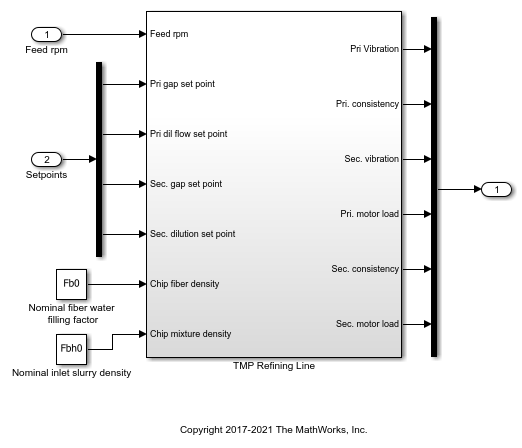# getInputIndex

Get index of an input element of an operating point specification

## Syntax

``index = getInputIndex(op,block)``
``index = getInputIndex(op,block,element)``

## Description

The `Inputs` property of an operating point specification is an array that contains trimming specifications for each model input. When defining a mapping function for customized trimming of Simulink® models, you can use `getInputIndex` to obtain the index of an input specification based on the corresponding block path.

When trimming Simulink models using optimization-based search, some applications require additional flexibility in defining the optimization search parameters. For such systems, you can specify custom constraints and a custom objective function. For complex models, you can define a mapping that selects a subset of the model states, inputs, and outputs to pass to the custom constraint and objective functions. For more information, see Compute Operating Points Using Custom Constraints and Objective Functions.

example

````index = getInputIndex(op,block)` returns the index of the input specification that corresponds to `block` in the `Inputs` property of operating point specification `op`.```

example

````index = getInputIndex(op,block,element)` returns the index of the specified `element` within an input specification for an input port that has a port width greater than `1`.```

## Examples

collapse all

```mdl = 'scdtmpSetpoints'; open_system(mdl) ```Create an operating point specification object for the model.

```opspec = operspec(mdl); ```

`opspec` contains specifications for the root-level input ports of the model.

```opspec.Inputs ```
```ans = u Known Min Max _____ _____ _____ _____ (1.) scdtmpSetpoints/Feed rpm 0 false -Inf Inf (2.) scdtmpSetpoints/Setpoints 0 false -Inf Inf 0 false -Inf Inf 0 false -Inf Inf 0 false -Inf Inf ```

Obtain the index of the specification in `opspec.Inputs` that corresponds to the Feed rpm input block.

```index1 = getInputIndex(opspec,'scdtmpSetpoints/Feed rpm') ```
```index1 = 1 1 ```

`index1(1)` is the index of the input specification object for the Feed rpm block in the `opspec.Inputs`. Since this input port is a scalar signal, `index1` has one row and `index1(2)` is `1`.

If an input port is a vector signal, you can obtain the indices for all of the elements in the corresponding input specification.

```index2 = getInputIndex(opspec,'scdtmpSetpoints/Setpoints') ```
```index2 = 2 1 2 2 2 3 2 4 ```

Each row of `index2` is the index for one element of the Setpoints input vector.

```mdl = 'scdtmpSetpoints'; open_system(mdl) ```Create an operating point specification object for the model.

```opspec = operspec(mdl); ```

`opspec` contains specifications for the root-level input ports of the model.

Obtain the index of the element that corresponds to the second signal in the Setpoints input vector.

```index1 = getInputIndex(opspec,'scdtmpSetpoints/Setpoints',2) ```
```index1 = 2 2 ```

You can also obtain the indices of multiple vector elements at the same time. For example, get the indices for the first and third elements of the Setpoints vector.

```index2 = getInputIndex(opspec,'scdtmpSetpoints/Setpoints',[1 3]) ```
```index2 = 2 1 2 3 ```

## Input Arguments

collapse all

Operating point specification or operating point for a Simulink model, specified as an `OperatingSpec`, `OperatingPoint`, or `OperatingReport` object.

Block path that corresponds to an input specification in the `Inputs` property of `op`, specified as a character vector or string that contains the path of a root-level input of a Simulink model.

To see all the blocks that have input specifications, view the `Inputs` property of `op`.

`op.Inputs`

Input element index, specified as a positive integer less than or equal to the port width of the input specified by `block`, or as a vector of such integers. By default, if you do not specify `element`, `getInputIndex` returns the indices of all elements in the selected input specification. For an example, see Get Index of Specified Input Element of Operating Point Specification.

## Output Arguments

collapse all

Input index, returned as a 2-element row vector when `element` is an integer, or a 2-column array when `element` is a vector. Each row of `index` contains the index for a single model input element.

The first column of `index` contains the index of the corresponding input specification in the `Inputs` property of `op`. The second column contains the element index within the input specification.

Using `index`, you can specify the input portion of a custom mapping for customized trimming of Simulink models. For more information, see the `CustomMappingFcn` property of `operspec`.

## Version History

Introduced in R2017a